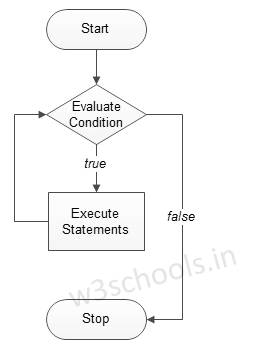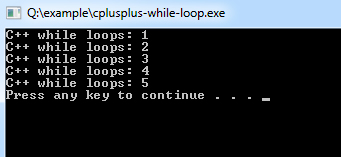## C++ Programming Tutorial Index

C++ `while` loop statement allows the same code block to be run repeatedly until a condition is met. This tutorial will teach you how to use the while loop in C++.

The `while` loop is the most basic loop in C++. It has a control condition and executes as long as the condition is `true`. In this loop, the condition of the loop is tested before the body of the loop is executed. That's why it is called an entry-controlled loop.

The basic format of the while loop statement is:

Syntax:

``````while (condition)
{
statement(s);
Incrementation;
}``````

Figure - Flowchart of while loop:Example C++ program to demonstrate the `while` loop:

Example:

``````#include <iostream>
using namespace std;

int main ()
{
/* local variable Initialization */
int n = 1, times=5;

/* while loops execution */
while( n <= times )
{
cout << "C++ while loops: " << n <<endl;
n++;
}
return 0;
}``````

Program Output: